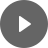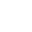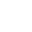11.1 Depreciation - StudyNinja
Select Page

HSC Standard Maths Resources

Browse: 1. Home  »  11. Depreciation & Loans »  11.1 Depreciation

11.1 Depreciation

Depreciation is the decline in value of an item over time. E.g. your car worth today may not be worth the same in a year time. The value, after taking account of depreciation, is called salvage value. There are two methods of depreciation; straight-line and declining balance. Straight-line depreciation reduces the value of item by constant amount every year.Example 1

I bought a car for \$25,000 which depreciates at 20% per year. What is its salvage value after 4 years and how much it depreciated?

Example 2

Continuing with same example of my car bought for \$25,000 and depreciating at 20%, which one of the following charts best shows salvage value of this car over time?Get access to 20 Mock Exams with over 700 exam-style questions for HSC Standard Maths.

You might find the following videos helpful related to this section:3C Appreciation and Inflation (1 of 4)

by Mr Bodgers (click to view channel)

Explains what appreciation is.3C Appreciation and Inflation (2 of 4)

by Mr Bodgers (click to view channel)

Completes an example on appreciation.3C Appreciation and Inflation (3 of 4)

by Mr Bodgers (click to view channel)

Explains what inflation is.3C Appreciation and Inflation (4 of 4)

by Mr Bodgers (click to view channel)

Completes an example on inflation.3E Declining Balance Depreciation (1 of 5)

by Mr Bodgers (click to view channel)

Explains the difference between straight line and declining balance methods of depreciation.3E Declining Balance Depreciation (2 of 5)

by Mr Bodgers (click to view channel)

Introduces the formula used for declining balance depreciation and explains the terminology used.3E Declining Balance Depreciation (3 of 5)

by Mr Bodgers (click to view channel)

Completes an example where you calculate the salvage value of a car.3E Declining Balance Depreciation (4 of 5)

by Mr Bodgers (click to view channel)

Completes an example where you calculate the purchase price of a vehicle given the salvage value.3E Declining Balance Depreciation (5 of 5)

by Mr Bodgers (click to view channel)

Completes an example where you calculate the rate of depreciation as a percentage given the salvage value and purchase price.The following is the type of questions you can expect in exam:

Study notes of this section and other resources can be accessed here:

Browse: 1. Home  »  11. Depreciation & Loans »  11.1 Depreciation